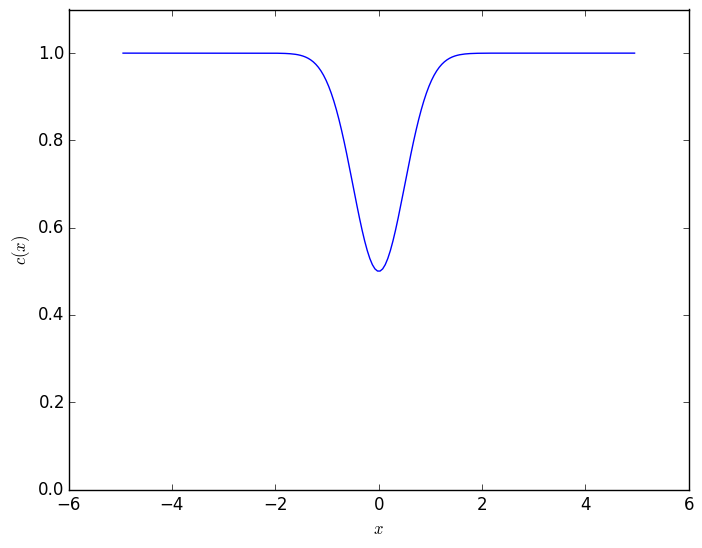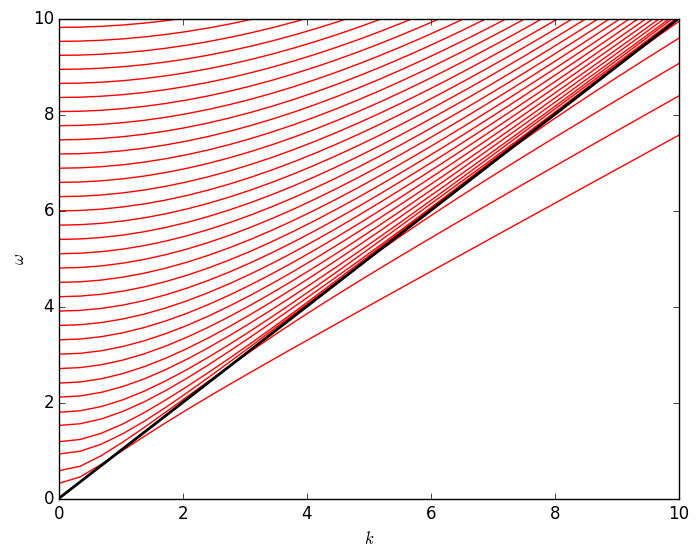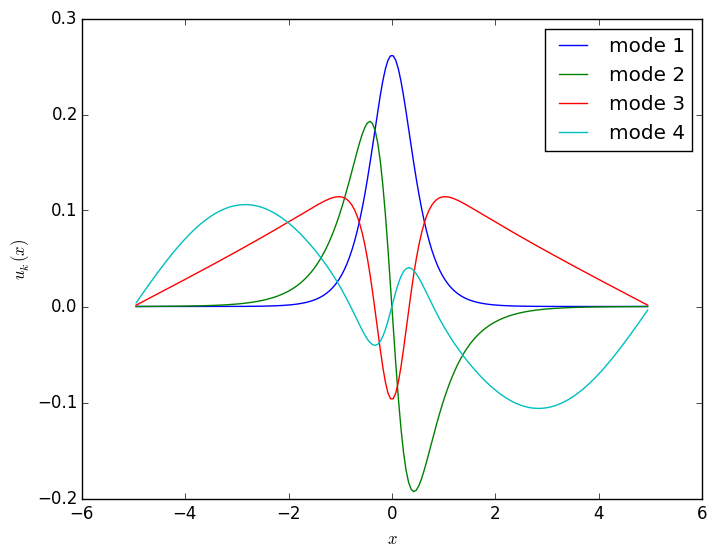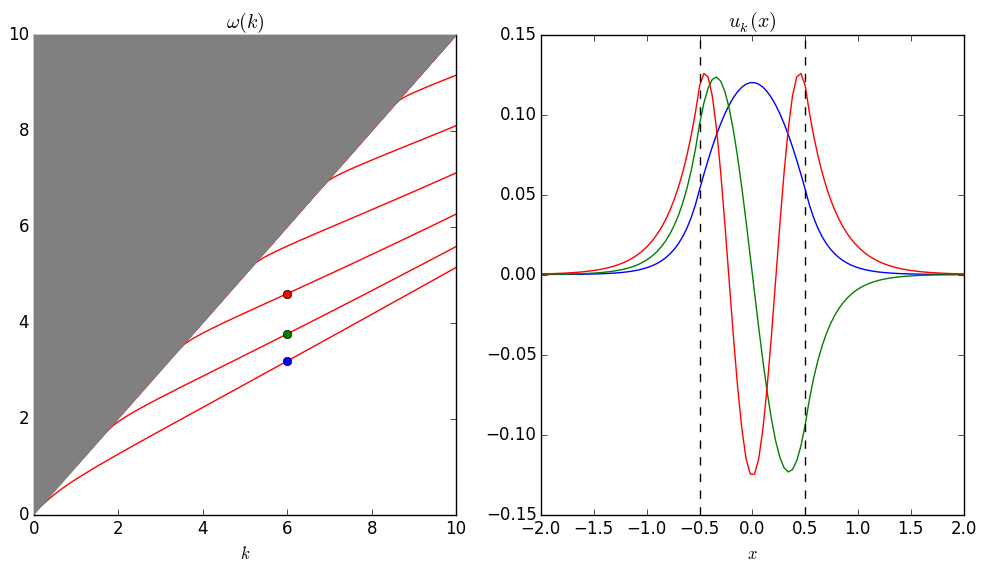In :
using PyPlot, Interact
WARNING: deprecated syntax "[a=>b, ...]" at /Users/stevenj/.julia/Interact/src/IJulia/setup.jl:68.

WARNING: deprecated syntax "[a=>b, ...]" at /Users/stevenj/.julia/Interact/src/IJulia/setup.jl:81.

WARNING: deprecated syntax "[a=>b, ...]" at /Users/stevenj/.julia/Interact/src/IJulia/setup.jl:196.

WARNING: deprecated syntax "{a=>b, ...}" at /Users/stevenj/.julia/Interact/src/IJulia/statedict.jl:5.

WARNING: deprecated syntax "{a=>b, ...}" at /Users/stevenj/.julia/Interact/src/IJulia/statedict.jl:10.

A waveguide eigenproblem¶

Let's compute the guided modes of $\nabla\cdot c(x) \nabla u = \ddot u$ in 2d $xz$, where we are looking for localized separable modes $u_k(x)e^{i(kz - \omega t)}$ in a region $0< c(x) \le 1$ surrounded by $c=1$. This gives us the eigenequation: $$\left[ -\frac{d}{dx} c(x) \frac{d}{dx} + k^2 c(x) \right] u_k = \omega(k)^2 u_k$$

In :
c = 0.5
c(x) = 1 - (1-c)*exp(-2x^2)
Out:
c (generic function with 1 method)

We will truncate our computational cell to $x \in [-L,L]$ with Dirichlet boundary conditions. For exponentially localized modes, the boundary conditions should be irrelevant as long as $L$ is big enough.

In :
L = 5
N = 200
dx = 2L / (N+1)
x = -L+dx:dx:L-dx
plot(x, [c(ξ) for ξ in x], "b-")
ylim(0,1.1); ylabel(L"c(x)"); xlabel(L"x")Out:
PyObject <matplotlib.text.Text object at 0x118087c90>
In :
# construct the (M+1)xM matrix D, not including the 1/dx factor
diff1(M) = [ [1.0 zeros(1,M-1)]; diagm(ones(M-1),1) - eye(M) ]

# grid points where derivative is evaluated
x = -L+dx/2:dx:L-dx/2
Out:
-4.975124378109452:0.04975124378109453:4.975124378109452
In :
function A(k)
D = diff1(N)/dx
return D' * diagm(Float64[c(ξ) for ξ in x′]) * D + k^2 * diagm(Float64[c(ξ) for ξ in x])
end
Out:
A (generic function with 1 method)
In :
# return p smallest eigenfrequencies of A(k)
function ω(k::Number,p)
return sqrt(eigvals(A(k))[1:p])
end
# for an array of k's, construct a matrix whose p columns are the p eigenfrequencies as a function of k
function ω(k::AbstractVector,p)
v = Array(Float64, length(k), p)
for i in 1:length(k)
v[i,:] = ω(k[i],p)
end
return v
end
Out:
ω (generic function with 2 methods)

Dispersion relation and guided modes¶

Now, let's plot the dispersion relation $\omega(k)$, along with the “light line” $\omega = k$ in black. Below the light line are a discrete set of lines corresponding to the guided modes (localized in the small-$c$ region). Above the light line would be continuum of solutions (the extended/non-localized modes) for $L \to \infty$, which here is discretized because of the finite $L$.

In :
k = linspace(0,10,30)
plot(k, ω(k,50), "r-")
plot(k, k, "k-", linewidth=2.0)
ylabel(L"\omega"); xlabel(L"k")
ylim(0,maximum(k))Out:
(0,10.0)

In the $L \to \infty$ limit, the lowest-$\omega$ guided mode (the “fundamental” mode) would lie below the light line for all $k > 0$, but here intersects $\omega=k$ at a nonzero $k$ because of the finite $L$: as $k \to 0$, we will see below that the fundamental mode becomes more and more delocalized, until it “sees” the boundaries and is messed up by the finite $L$. In contrast, even for $L \to \infty$, all of the subsequent guidede modes have a low-$\omega$ cutoff where they intersect the light line at $k > 0$.

Let's now plot the mode profiles $u_k(x)$ for the first few modes, with a slider control (via @manipulate in the Interact package included above) so that we can vary $k$.

In :
f = figure()
nmodes = 4
@manipulate for k in 0:0.1:10
withfig(f) do
λ,U = eig(A(k))
for j = 1:nmodes
u = U[7,j] < 0 ? -U[:,j] : U[:,j] # make sure we choose a consistent sign as k changes
plot(x,u)
xlabel(L"x"); ylabel(L"u_k(x)");
legend(["mode $n" for n in 1:nmodes]) end end end WARNING: apply(f, x) is deprecated, use f(x...) instead in lift at /Users/stevenj/.julia/Reactive/src/Reactive.jl:296 in include_string at loading.jl:97 in execute_request_0x535c5df2 at /Users/stevenj/.julia/IJulia/src/execute_request.jl:140 in eventloop at /Users/stevenj/.julia/IJulia/src/IJulia.jl:123 in anonymous at task.jl:340 Out:WARNING: apply(f, x) is deprecated, use f(x...) instead in init_comm at /Users/stevenj/.julia/Interact/src/IJulia/setup.jl:71 in metadata at /Users/stevenj/.julia/Interact/src/IJulia/setup.jl:80 in execute_request_0x535c5df2 at /Users/stevenj/.julia/IJulia/src/execute_request.jl:183 in eventloop at /Users/stevenj/.julia/IJulia/src/IJulia.jl:123 in anonymous at task.jl:340 Another wave equation¶ Now, for fun, let's try another wave equation$c^2\nabla^2 u = \ddot{u}$, again in 2d with an$x$-dependent$c(x)$, leading to the reduced eigenproblem: $$\left[ -\frac{d^2}{dx}^2 + k^2 \right] u_k = \omega^2 c^{-2} u_k ,$$ where for computational convenience we have written it in the form of a generalized eigenproblem$\hat{A}_k u_k = \omega^2 \hat{B} u_k$, with an operator$\hat{B} = c^{-2}$on the right-hand side. When we discretize it, this will lead naturally to a generalized Hermitian eigenproblem$Ax = \lambda Bx$with symmetric-definite matrices$A$and$B$. In this case, we will again use an$x \in [-L,L]$truncated computational cell, but this time we will choose a piecewise-constant$c(x) = c_1 < 1$for$|x| < h/2$and$c(x) = c_0 = 1\$ otherwise.

In :
function AB(k, h, c, L, N)
dx = 2L / (N+1)
x = -L+dx:dx:L-dx
c = Float64[ abs(ξ) < 0.5*h ? c : 1 for ξ in x]
D = diff1(N)/dx
A = D'*D + k^2 * I
B = diagm(c.^(-2))
return (A,B)
end
Out:
AB (generic function with 2 methods)
In :
figure(figsize=(12,6))
subplot(1,2,1)
k = linspace(0,10,60)
plot(k, vcat([sqrt(eigvals(AB(κ,1,0.5,20,1000)...)[1:20])' for κ in k]...), "r-")
fill_between(k, k, ones(k)*maximum(k), zorder=10, color="gray")
title(L"\omega(k)"); xlabel(L"k")
ylim(0,maximum(k))
λ, U = eig(AB(6,1,0.5,20,1000)...)
for j = 1:3
plot(, [sqrt(λ[j])], "o")
end
subplot(1,2,2)
Δx = 2*20 / (1000+1)
xs = -20+Δx:Δx:20-Δx
for j = 1:3
u = U[490,j] < 0 ? -U[:,j] : U[:,j]
plot(xs, u)
end
plot([-0.5,-0.5],[-0.15,0.15],"k--")
plot([+0.5,+0.5],[-0.15,0.15],"k--")
xlabel(L"x"); title(L"u_k(x)")
xlim(-2,2); ylim(-0.15,0.15)
savefig("waveguide-modes-plot.pdf")In [ ]: